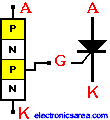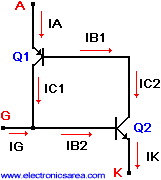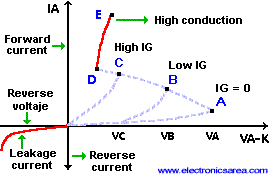The SCR (Silicon Controled Rectifier)

The SCR is a 4 layers semiconductor device which behaves almost as an ideal switch. Thyristor symbol and structure are shown at right. A = anode, G = gate and K = cathode.Basic Operation of a SCR

To understand the SCR operation, the following picture shows an equivalent circuit.• An IG current is applied to the G terminal (base terminal of transistor Q2 and collector terminal of transistor Q1) then IC2 = ßIB2, but IC2 = IB1.
• IB1 is the base current of the Q1 transistor and it makes a collector current flow (IC1).
• The IC1 is applied to the base of the transistor Q2 (IB2), causing more collector current in Q2 (IC2). The IC2 is the same IB1 current that flows on the base of Q1, and so on.

This regenerative process is repeated until Q1 and Q2 gets saturated causing the thrystor to turn on.

The parameters of the SCR are:

• VRDM: Maximum reverse voltage (VG = 0)
• VFOM: Maximum direct voltage (VG = 0)
• IF: Maximum allowed direct current
• PG: Maximum dissipation of power between gate and cathode.
• TGV-IGT: Maximum voltage – current required in the gate (G) to trigger the SCR
• IH: Minimum anode current required to keep the thyristor on its ON state
• Dv/dt: Maximum voltage variation without triggering.
• Di/dt: Maximum accepted current variation before destroying the thyristor.

Note: dv/dt, di/dt: See parameters of SCR in thyristor on DC.

Characteristic curve of the SCR

The picture below shows the relation between the switching voltage and the gate current.When the SCR is reversed biased; it behaves like a common diode (see the leakage current feature).

The SCR in its direct bias region also behaves like a common diode. (When it has been already activated (On)). See points D and E. (high conduction).  For high gate current (IG) values (see point C), the anode-cathode voltage is lower (VC).

If the IG decreases, the anode-cathode voltage increases. (See points B and A, and anode-cathode voltage VA and VB). In conclusion, if we reduce the gate IG current, the anode-cathode voltage will tend to increase before the SCR is ON.

•
•
•
•
•
•
•
•
•
•
•
•
•
•
•
•
•
•